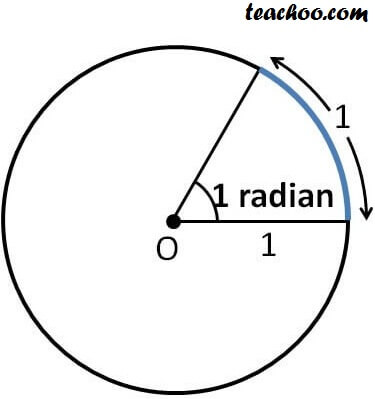Chapter 3 Class 11 Trigonometric Functions
Concept wise

In higher classes, we don’t use degree to measure angles

We define radian in terms of length of arc :

Angle subtended by an arc of 1 unit in a circle of radius 1 unit is 1 radian .

In other words,

• If length of arc is 1 unit
• & Radius = 1 unit
• Then, angle at center = 1 radianNote: In θ = l/r i.e. length of arc formula,

Learn in your speed, with individual attention - Teachoo Maths 1-on-1 Class

### Transcript

In higher classes, we don’t use degree to measure angles We use radians. We define radian in terms of length of arc: Angle subtended by an arc of 1 unit in a circle of radius 1 unit is 1 radian. In other words, If length of arc is 1 unit & Radius = 1 unit Then, angle at center = 1 radian Note: In θ = l/r i.e. length of arc formula, Angle is measured in radians